# Identify the oxidizing agent and the reducing agent for each reaction: Drag the appropriate labels to...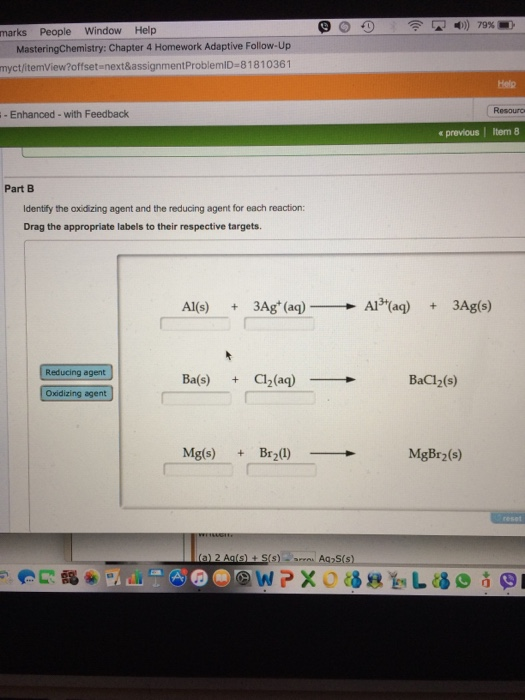Identify the oxidizing agent and the reducing agent for each reaction: Drag the appropriate labels to their respective targets.

Reducing agent (RA) is the one that get oxidised ie, the oxidation number increases.

Oxidising agent (OA) is the one that get reduced ie, the oxidation number decreases.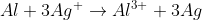The oxidation state of Al goes from 0 to +3, so itsthe RA.

Ag oxidation state decreases from +1 to 0, so its the OA.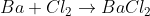Oxidation state of Ba increases from 0 to +2, so its the RA.

Oxidation state of Cl decreases from 0 to -1, so its the OA.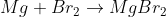Oxidation state of Mg increases from 0 to +2, so its the RA.

Oxidation state of Br decreases from 0 to -1, so its the OA.

#### Earn Coin

Coins can be redeemed for fabulous gifts.

Similar Homework Help Questions
• ### Part A: Label each reactant as the reducing agent, oxidizing agent, or neither. Drag the appropriate...

Part A: Label each reactant as the reducing agent, oxidizing agent, or neither. Drag the appropriate labels to their respective targets. Oxidizing agent Oxidizing agent Neither N2 + 3H2 ---------> 2NH3 Reactants: [] [] 2N2 + O2 ---------> 2N2O Reactants: [] [] Part B: Here is a more complex redox reaction involving the permanganate ion in acidic solution. Label each reactant as the reducing agent, oxidizing agent, or neither. Drag the appropriate labels to their respective targets. Oxidizing agent Reducing...

• ### Identify Oxidizing and Reducing Agents

Part A:Label each reactant as the reducing agent, oxidizing agent, or neither.Drag the appropriate labels to their respective targets.Oxidizing agent Oxidizing agent NeitherN2 + 3H2 ---------> 2NH3Reactants: [] []2N2 + O2 ---------> 2N2OReactants: [] []Part B:Here is a more complex redox reaction involving the permanganate ion in acidic solution. Label each reactant as the reducing agent, oxidizing agent, or neither.Drag the appropriate labels to their respective targets.Oxidizing agent Reducing agent Neither5NO2^- + 6H^+ + 2MnO4^- ------> 5NO3^- + 2Mn^2+ +3H20Reactants:...

• ### Submit Part B Identify the oxidizing agent and the reducing agent in each reaction below. Drag...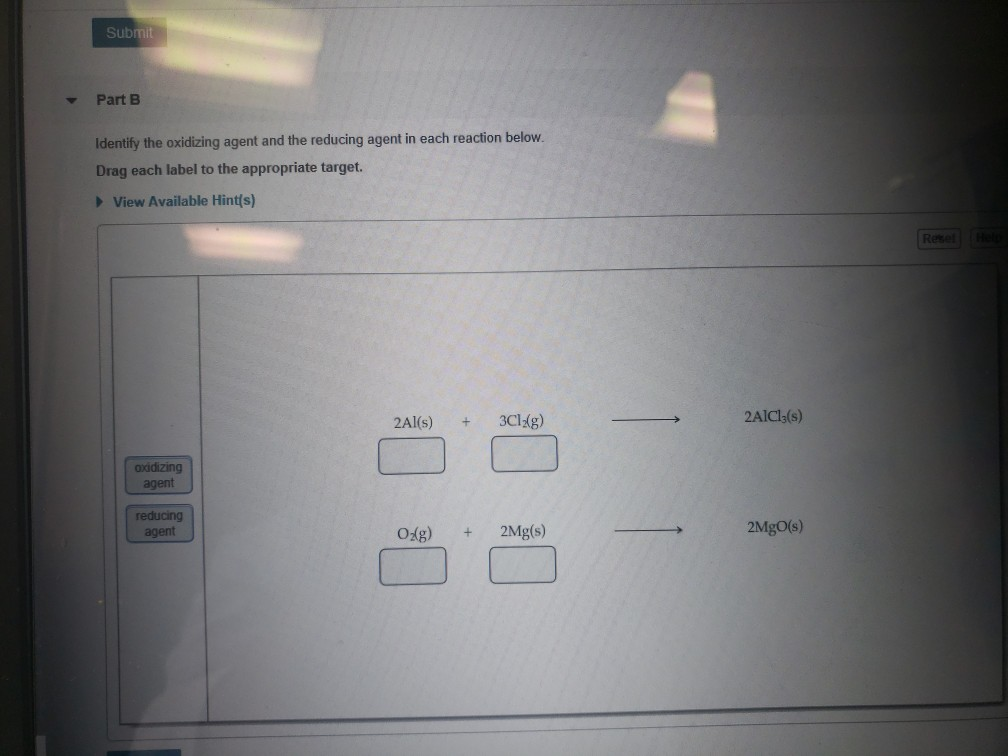Submit Part B Identify the oxidizing agent and the reducing agent in each reaction below. Drag each label to the appropriate target. View Available Hint(s) Reselle 2Al(s) + 3Cl2(g) 2AlCl3(s) addizing agent reducing agent Odg) + 2Mg(s) 2MgO(s)

• ### drag the appropriate labels to their respective targets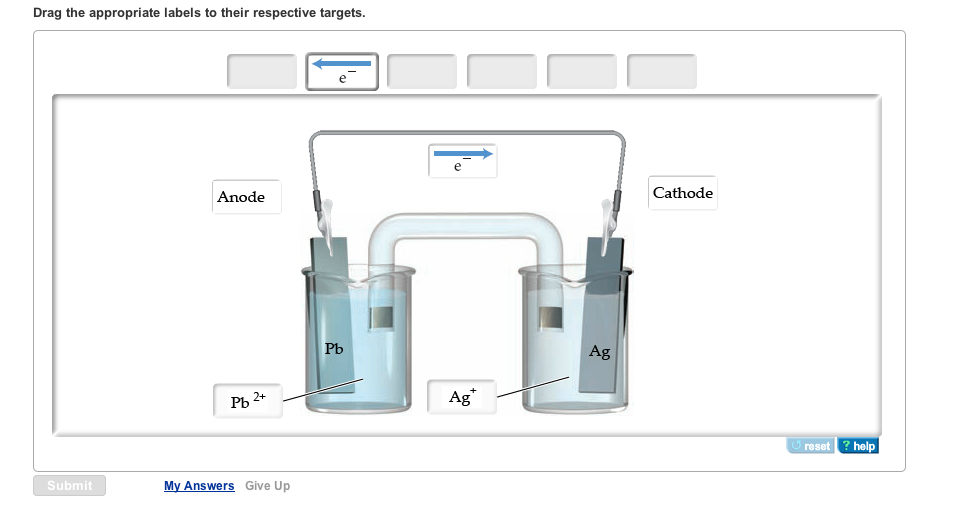Drag the appropriate labels to their respective targets. Indicate the half-reaction occurring at Anode. Express your answer as a chemical equation. Identify all of the phases in your answer. Indicate the half-reaction occurring at Cathode. Express your answer as a chemical equation. Identify all of the phases in your answer. 2ClO2(g) + 2I- (aq) 2ClCO2- (aq) + I2(s) Drag the appropriate labels to their respective targets. Indicate the half-reaction occurring at Anode. Express your answer as a chemical equation. Identify...

• ### Identify which is the reducing agent and which is the oxidizing agent in the reaction of...

Identify which is the reducing agent and which is the oxidizing agent in the reaction of benzophenone with sodium borohydride and explain your reasoning, ie. show the changes in oxidation states (best to give a drawing as per the introduction)?  Identify which is the reducing agent and which is the oxidizing agent in the reaction of benzophenone with sodium borohydride and explain your reasoning, ie. show the changes in oxidation states (best to give a drawing)?

• ### Part A Identify the oxidizing agent and the reducing agent in the reaction Cus+H, Ou+H,S. Match...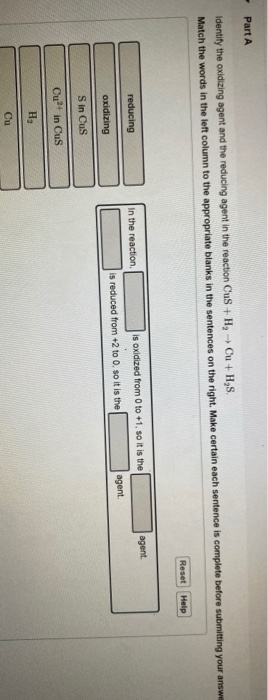Part A Identify the oxidizing agent and the reducing agent in the reaction Cus+H, Ou+H,S. Match the words in the left column to the appropriate blanks in the sentences on the right. Make certain each sentence is complete before submitting your answer Reset Help agent. reducing in the reaction is oxidized from 0 to +1, so it is the is reduced from +2 to 0. so it is the agent. oxidizing Sin Cus Cut in Cus

• ### Identify the species oxidized, the species reduced, the oxidizing agent and the reducing agent in the...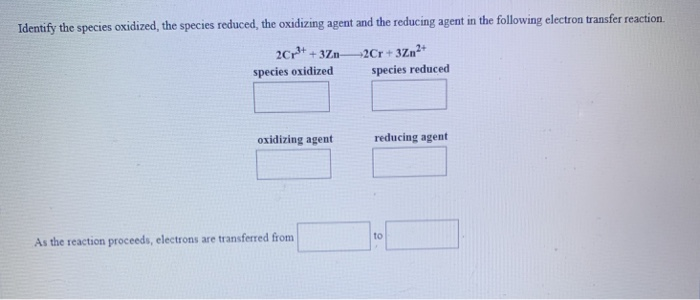Identify the species oxidized, the species reduced, the oxidizing agent and the reducing agent in the following electron transfer reaction. 2Cr3+ + 3712Cr + 3Zn2+ species oxidized species reduced oxidizing agent reducing agent As the reaction proceeds, electrons are transferred from Identify the species oxidized, the species reduced the oxidizing agent and the reducing agent in the following electron transfer reaction. Hg2+ + SnHg+Sn2+ species oxidized species reduced oxidizing agent reducing agent As the reaction proceeds, electrons are transferred from

• ### Drag the appropriate labels to their respective targets. Note: not all labels will be used.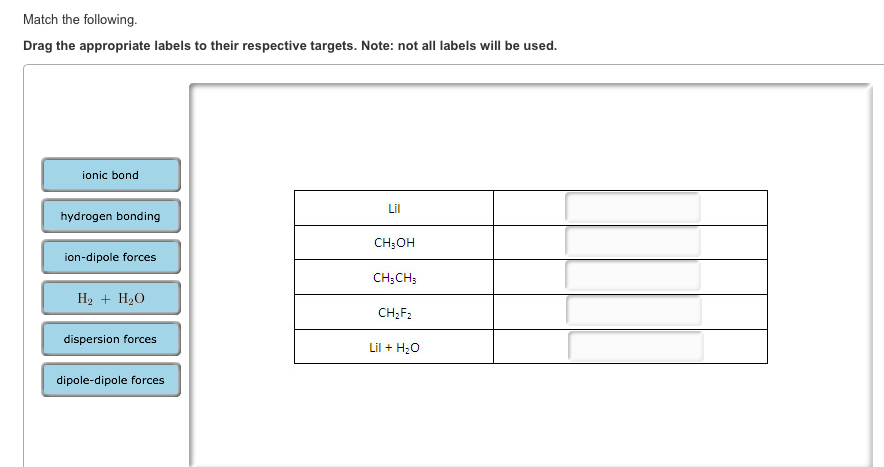Match the following. Drag the appropriate labels to their respective targets. Note: not all labels will be used. Match the following. Drag the appropriate labels to their respective targets. Note: not all labels will be used. Match the following. Drag the appropriate labels to their respective targets. Note: not all labels will be used.

• ### Identify the reducing agent and the oxidizing agent in this reaction. Fe2O3 + 2Al --> Al2O3...

Identify the reducing agent and the oxidizing agent in this reaction. Fe2O3 + 2Al --> Al2O3 + 2Fe

• ### Identify the oxidizing agent and the reducing agent in each of the following

Identify the oxidizing agent and the reducing agent in each of the following. (Type your answer using the format Al for aluminum, [H]+ for H+ and [O]2- for O2-. Do notinclude state symbols in the answers.) (a) 8 H+(aq) + Cr2O72-(aq) + 3SO32-(aq) 2 Cr3+(aq) + 3 SO42-(aq) + 4 H2O(l) oxidizing agent reducing agent (b) 2 MnO4-(aq) + 10Cl-(aq) + 16H +(aq) 5 Cl2(g) + 2 Mn2+(aq) + 8 H2O(l) oxidizing agent reducing agent